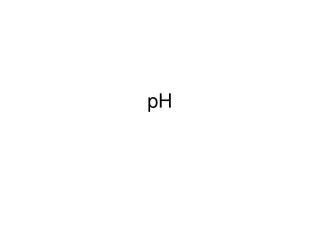DownloadDownload PresentationDefinitions and Formulas

# Definitions and Formulas

Télécharger la présentation## Definitions and Formulas

- - - - - - - - - - - - - - - - - - - - - - - - - - - E N D - - - - - - - - - - - - - - - - - - - - - - - - - - -
##### Presentation Transcript

1. pH

2. Definitions and Formulas • The pH scale is defined as the negative log of the concentration of H+: pH = -log[H+] • The pOH scale is defined as the negative log of the concentration of OH-, [OH-]. pOH = -log[OH-] • With this scale, calculating the pOH can be done in the same manner as the pH scale. pH + pOH = 14

3. Examples What is the pH of a solution that contains 25 g of hydrochloric acid (HCl) dissolved in 1.5 L of water?

4. Quiz • Determine the pH of a .0034 M HNO3 solution. • Determine the pOH of the solution in #1. • What is the pH of a 2.4 x 10-5 M HCl solution?

5. Auto-Ionization of Water In water, Kc= [H+][OH-] 55.5 M [OH-]= Kw[H30+]= Kw [H30+] [OH-] ion product constant for water  Kc (55.5M) = [H+][OH-] = Kw  Kw= [H30+] [OH-]  Kw= 10 -14 Example: What is the hydroxide ion concentration in an aqueous solution that is 4.0 x 10-5 M H30+? [OH-] = (1.0 x 10-14) (4.0 x 10-5) =2.5 x 10-10 M

6. Practice What is the hydronium ion concentration in an aqueous solution containing 3.5 x 10-7 OH-? [H30+] = (1.0 x 10-14) (3.5 x 10-7) =2.9 x 10-8 What is the hydroxide ion concentration in an aqueous solution containing 8.34 x 10-12 H30+ ? [OH-] = (1.0 x 10-14) (8.34 x 10-12) =1.20 x 10 -3

7. Strong Acids • dissociate completely in water to form hydronium ion(s) • Examples • HCl • HNO3 • H2SO4

8. Strong Bases • dissociate completely in water to form hydroxide ions • Examples: • NaOH • KOH • Ca(OH)2